## Solve the quadratic equation by factoring. 2x^2+5x+3=0

Question

Solve the quadratic equation by factoring. 2x^2+5x+3=0

in progress 0
2 months 2021-07-24T17:39:34+00:00 2 Answers 2 views 0

-0.33 repeating

Step-by-step explanation:

2x^2=4x

4x+5x+3=0

combine like terms

9x + 3 = 0

subtract 3 from both sides

9x = -3

divide 9 both sides to get the variable by itself

x = -0.33 repeating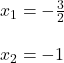Step-by-step explanation:

the general fromula is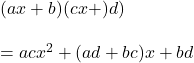so we need that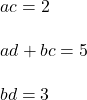for ac = 2

we only have 2 and 1

so let’s say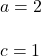so we now have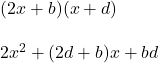and for bd = 3

we only have 3 and 1

but we need to

2d +b = 5

so we can have

b=3

and

d=1

so the factorized form is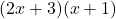so we come back to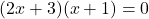so here we have two cases

Case 1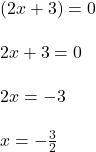Case 2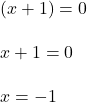so those are our solutions